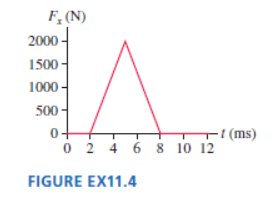# Problem: What is the impulse on a 3.0 kg particle that experiences the force shown in Figure EX11.4?

###### FREE Expert Solution

Impulse;

$\overline{){\mathbf{J}}{\mathbf{=}}{{\mathbf{\int }}}_{{\mathbf{t}}_{\mathbf{0}}}^{{\mathbf{t}}_{\mathbf{f}}}{\mathbf{F}}{\mathbf{\left(}}{\mathbf{t}}{\mathbf{\right)}}{\mathbf{d}}{\mathbf{t}}}$

This implies that the impulse is the area under the curve.

94% (280 ratings)###### Problem Details

What is the impulse on a 3.0 kg particle that experiences the force shown in Figure EX11.4?